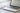## Multi value arrays: How to create in PowerShell really easy

Hello together, in my latest activity as an consutlant I encountered following issue: I got a objects with two meta data. In my example it was a milestone with an link to the milestone activity. Since it were more than 30 items, I didn’t want to work with PSObjects. I was searching for a solution to create multi value arrays with little code as possible. If I would work with PSObjects, it would blow up my code. In this article, I will show you how you can create “normal” arrays and also how you can enhance this arrays, to multi value arrays.

## Create Standard PowerShell Arrays in PowerShell

A standard array is set up like this:

``\$array = @("Value1","Value2")``

You can also write it like this:

```\$Array = @(
"Value1"
"Value2"
)```

So you can get the single values, by putting in the index number of it in square brackets – like `\$Array` for the first value and `\$Array` for the second value. With [-1] you can get the last value of the array.

### Add Value to Standard PowerShell Arrays

You can add new lines in the array like this:

``\$Array.Add("Value3")``

### Remove Value from Standard PowerShell Arrays

You can remove the lines of an array like this:

``\$Array.Remove("Value2")``

## Create multi value arrays in PowerShell

For creating a multi value array, we have to inject a hashtable to each array line.

A hashtable looks like this:

``@{KEY="VALUE"; KEY2 = "VALUE2"; KEYn = "VALUEn"}``

So your multi value array will look like this:

```\$Array = @(
@{ Key1=("Value1"); Key2=("Value2")}
@{ Key1=("Value3") ;Key2=("Value4")}
)```

For my example it looks like this:

```\$Array = @(
)```

As you can see, you can also get the single values of each line in array:

## Add Value to mutli value Arrays in PowerShell

You can add a value to a multidimensional array in PowerShell like this:

``\$Array.Add( @{ Displayname=("Ad-equum"); Link=("https://www.ad-equum.de/")} )``

## Remove Value from multi value arrays in PowerShell

Removing a value from a multi value array in PowerShell is more complicated. You have to redefine the array, by filtering out the value, which you don’t want in your array:

``\$Array = \$Array | Where-Object {\$_.displayname -ne "Ad-equum"}``

## Conclusio

If you want to create a multi vlaue array in PowerShell, the only thing you have to do is to inject a hashtable to each line. I hope, that I have saved you a ton of work 🙂